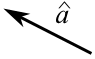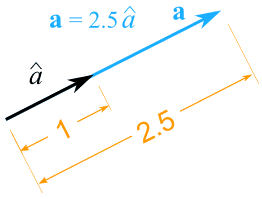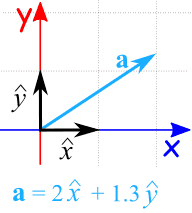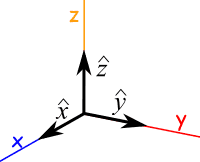# Unit Vector

A vector has magnitude (how long it is) and direction:## Unit Vector

A Unit Vector has a magnitude of 1:The symbol is usually a lowercase letter with a "hat", such as:(Pronounced "a-hat")

## Scaling

A vector can be "scaled" off the unit vector. Here vector a is shown to be 2.5 times a unit vector. Notice they still point in the same direction:## In 2 Dimensions

Unit vectors can be used in 2 dimensions:Here we show that the vector a is made up of 2 "x" unit vectors and 1.3 "y" unit vectors.

## In 3 Dimensions

Likewise we can use unit vectors in three (or more!) dimensions: